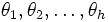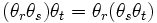# History of groups

Jump to: navigation, search
This is a survey article related to:group
View other survey articles about group

This article is about the history and development of the notion of group, till the stage of the formal definition that we study these days in textbooks. The development of group theory beyond that point is not discussed here.

For a list of historical definitions of group, refer historical definitions of group.

## Groups before groups

People have been studying some particular groups long before the concept of a group was first formally mooted. Trivial examples are the rational numbers, the complex numbers, and the real numbers (although a proper understanding of the concept of a real number happened only in the nineteenth century, after the concept of group had been mooted). Some more nontrivial kinds of groups that had been studied before groups were formally proposed:

• Groups of rotations, translations and dilatations, and other geometric symmetries (a non-Abelian example)
• Modular arithmetic (an Abelian example)

Before the concept of group was formally mooted, there were a number of limitations in the way mathematicians thought of groups.

### Lack of clear definitions

• Lack of closure: People talked of certain symmetries of a structure in order to study symmetry-invariance, but it was not usually typical to saturate or close the symmetries to form a collection of symmetries closed under taking inverses and compositions. For instance, we now consider the group of all Euclidean motions, or of all permutations, but from a practical viewpoint, proving symmetry-invariance may only require one to take a generating set.
• No explicit statement of associativity: The notions of associativity and commutativity had not been encoded universally, though mathematicians who dabbled with groups used the associativity.
• No explicit statement or assumption of identity element and inverses: The existence of identity elements and inverses was also not usually stated, or even assumed.

### Trapped in a concrete world

The concept of groups, prior to an explicit definition of groups, had the following traps:

• A group was not treated as a set with an arbitrarily prescribed binary operation; rather, it was as if the multiplication existed from before in some natural fashion (for instance, as composition of permutations).
• Isomorphic groups arising in different contexts were not recognized as being the same thing. Even in situations where they were so recognized, this recognition was not encoded formally and was used more as an intuitive guide.

### Devoid of set-theoretic notation

Much of the early development of group theory happened at a time when the conventions of naive set theory were not developed. The notation was ad hoc and clumsy, and this impeded the development as well as the concise formulation of ideas.

For instance, a group was treated as a listing or enumeration of symbols -- this did not cover reasonably the infinite groups. Much of the initial development of groups was for finite groups.

## A group of transformations

### The name, and closure

Time: 1770-1800

The term group initially arose as the notion of a group of transformations. Here group means collection or set in the loose sense of the word. The idea was to consider a set and certain transformations from that set to itself that were invertible.

Lagrange first studied groups of permutations in the 1770s. Lagrange wanted to determine whether polynomial equations of degree greater than four could be solved by means of radicals. He observed that solutions for the cases$n = 3, n = 4$ involved reducing the polynomial equation to an auxiliary equation of lower degree, and wanted to determine whether the same can be done for$n = 5$. For the purpose, he studied the group of symmetries between the roots of a polynomial equation.

Nonetheless, Lagrange did not explicitly state an axiomatization, nor did he bother to close his groups under multiplication and inverses.

Ruffini, in an attempt to prove the unsolvability of the quintic equation, first introduced notions of groups of permutations that were closed and were truly groups in the sense that we talk of now, and he also introduced his equivalents of cyclic groups.

Cauchy took these ideas further, and introduced the notation of powers that made certain ideas in group theory clearer. Abel gave an accepted proof of the unsolvability of the quintic, using the ideas of permutation groups.

### The breakthroughs by Galois

Time: 1800-1850

Galois studied the permutations of roots of an equation, along the same lines as Abel or Ruffini. His breakthrough insight was that the internal structure of the group of permutations controlled the solvability of the equation. The way Galois was studying groups, he was still viewing groups of permutations, and not abstract groups the way they are viewed now. However, he was looking at certain structural aspects of these groups that are isomorphism-invariant in our modern sense and independent of the particular action. This led him to look at normal subgroups, quotients and composition series, and the concept of a solvable group.

By now:

• A group usually meant a finite group acting as symmetries on a finite set. Thus, the group was still firmly viewed as acting on something. However, aspects of the internal structure of groups had started receiving more attention.
• Groups were now assumed to be closed under composition, and to satisfy laws like cancellation and associativity.

Despite this, many of the people who understood the importance of Galois's work, including Liouville, failed to realize the importance of abstracting and isolating the group concept. Betti and Serret made further contributions into providing rigor to the ideas introduced by Galois.

## Abstract development of groups

### A first attempt at definition by Cayley

Time: 1854

Cayley attempted the first general definition of a group, in his paper On the theory of groups, as depending on the symbolic equation$\theta^n = 1$. This definition is not precisely the same as the modern definition, though it is equivalent. Cayley states:

A set of symbols$1, \alpha, \beta, \dots$, all of them different, and such that the product of any two of them (no matter in what order), or the product of any one of them into itself, belongs to the set, is said to be a group. These symbols are not in general convertible (commutative) but associative, it follows that if the entire group is multiplied by any one of the symbols, either as further or nearer factor (left or right), the effect is simply to reproduce the group.

On the positive side:

• Cayley's definition talks very clearly of the closure property of groups. Cayley's groups are closed under the binary operation.
• Cayley also explicitly stated the associativity assumption.
• While Cayley did not use the identity element and inverses in defining the concept of group,he used an equivalent criterion -- the left and right cancellation. Further information: Associative quasigroup implies group
• Cayley dealt with his group as a set with binary operation, or something very close to that. He did not try to pre-determine where the product came from, but rather, tried to set axiomatic conditions the product must satisfy.

On the negative side:

• It implicitly assumed an enumeration of the symbols, possibly restricting the context to finite groups. This is best understood in terms of the lack of a universally established standard of set-theoretic notation.
• It did not clearly talk of a set with a binary operation. Rather, it assumed a prior sense in which multiplication has been defined, and simply demands the closure of the set under this operation. This, again, can be attributed to the absence of an established standard of set-theoretic notation.
• It did not state the identity element (neutral element) and inverse element axioms explicitly.
• It did not explicitly define isomorphism of groups and isomorphic groups, though Cayley did make observations related to this -- like the group of integers mod$n$ and the group of$n^{th}$ roots of unity, are in every respect similar.

### Jordan-Holder theorem

Time: 1860-1880

Jordan first proved the version of the Jordan-Holder theorem for permutation groups, and Holder then generalized it to abstract groups. Around this time, the structure of groups in the abstract had started receiving more attention, and people had started seeing the advantages in proving results for abstract groups.

### The geometric perspective

Time: 1870-1900

Although it had been known that infinite groups occurred in geometry, this had not been studied systematically or given much importance till the second half of the nineteenth century. It was Klein who introduced the Erlanger Program (in 1872) whose central thesis was that geometry is the study of groups of transformations and those properties that remain invariant under the transformations. Thus, the classical Euclidean geometry could be viewed as the study of those properties of Euclidean space that remained invariant under the group of Euclidean motions. This suggested that to understand geometry, it was important to study the infinite groups occurring in geometry, and the way they acted on spaces.

### The analytic perspective

Time: 1880-1900

Sophus Lie tried to do for differential equations what Galois did for polynomial equations. He considered groups of symmetries between the solutions to a differential equation. These groups are very different from permutation groups on finite sets: they are infinite, and there is a topology on them: a notion of continuity and proximity between group elements. Lie's work led to the notion of Lie group. Lie further introduced a relatively more tractable algebraic object called Lie algebra, and related the properties of a Lie group to the properties of its Lie algebra.

### More formal definitions

Time:1875-1890

Heinrich Weber attempted a definition of group in a paper in 1882. Weber's definition was:

A system$G$ of$h$ arbitrary elements$\theta_1, \theta_2, \dots, \theta_h$ is called a group of degree$h$ if it satisfies the following conditions:

I. By some rule which is designated as composition or multiplication, from any two elements of the same system one derives a new element of the same system.
II. It is always true that$(\theta_r \theta_s)\theta_t = \theta_r(\theta_s\theta_t)$.

III. From$\theta \theta_r = \theta \theta_s$ it follows that$\theta_r = \theta_s$.

This definition is not complete -- a dual statement to III. is needed that allows for right cancellation. With this dual statement, Weber has defined a cancellative semigroup. It turns out that in the finite case, this is equivalent to the definition of group. Further information: Associative quasigroup implies group

von Dyck, Klein's assistant, introduced the formal definition of group that is universally accepted now: a set with a binary operation and some further operations/conditions (associativity, identity elements, and inverses). His main insight was the explicit introduction of axioms for the identity element and inverses. von Dyck also argued for the use of presentations to describe groups, and he introduced the explicit notion of isomorphism of groups.

## Today

Today, when group theory is taught in undergraduate curricula, it is inevitable (after a bit of motivation) to plunge into the modern definition of groups, and it may seem strange to us that people who dabbled with groups for a long time did not see this definition as a useful one to work with. But it should be remembered that mathematics has come a long way in abstraction. Today, when a new concept is introduced, there are enough mathematicians who will work towards obtaining a general framework in which it fits. We also have a large number of well-tested languages and formalisms (including category theory, universal algebra, model theory, and a number of more specific languages) that address questions arising when one is trying to abstract out a concept.